Enter forum description here ...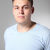+35
Completed

###fsolve()

Denys Dutykh 7 years ago updated by Pavel Holoborodko 4 years ago

Another very useful functionality would be to have a multi-precision analogue of fsolve(). In my opinion, it would seriously increasy the number of potential clients of the MC Toolbox...+8

###Optimization toolbox

Did 7 years ago updated by Pavel Holoborodko 7 years ago

Support for Optimization and Global Optimization toolboxes would be great.

(To avoid licence conflicts, Advanpix could run these arbitrary precision extensions only if ones has the licence for the "normal" toolbox.)+5
Completed

###Sparse Matrices Rudimentary Support

Pavel Holoborodko 7 years ago updated 7 years ago

Brief plan for ongoing development.

1. Introduce multiprecision sparse matrix type (provide smooth integration with MATLAB and toolbox itself).

2. Conversion from/to built-in 'double' sparse matrices.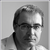+3
Completed

###Adaptive Gauss-Kronod quadrature: quadgk()

Viktor Witkovsky 7 years ago updated by Pavel Holoborodko 5 years ago

Although an algorithm for multiprecision computation of Gauss-Kronod nodes and weights is available, I suggest to implement multiprecision version (quadruple precision) of the algorithm quadgk.+2
Completed

###randn()

Denys Dutykh 7 years ago updated by Pavel Holoborodko 7 years ago

I would suggest to add a function randn() which generates pseudo-random numbers according to the normal distribution.

In several cases it happened to me that I needed to generate a randomly perturbed initial condition to test the sensitivity of the solution by performing then extended multi-precision computations.Pavel Holoborodko 7 years ago

Today we have added mp.randn to the toolbox (Windows).

Linux & Mac OS X versions will be updated shortly.

Thank you for the suggestion.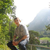+2
Completed

###Optimized array manipulation operations

Ilya Tyuryukanov 6 years ago updated by Pavel Holoborodko 5 years ago
I think it would be nice to speed up the logical indexing and other such array manipulation commands, because they are critical for many matrix- and vector-based algorithms. As for now, e.g. X(1:5) or X.' would take about 20 times more time if X is mp compared to double X (correct me if I'm wrong, or there's a way for speed increase). I know from the blog post that it was even slower earlier, but still such operations are very often and hence worth of optimization (IMHO).+1
Under review

###It would be fantastic if `quadeig` is supported.

kuanxu33 9 months ago updated by Pavel Holoborodko 8 months ago

Only `polyeig` is equipped with MP toolbox.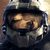+1
Thanks

### Accumulation of double precision vector

dattorro 1 year ago updated by Pavel Holoborodko 1 year ago

Quadruple precision floating-point accumulation of a double precision vector  p  is emulated numerically in Matlab:

```function t = orosumvec(p, recurs)
x = cumsum(p);
z = x - p;
u = (z - x) + p;
v = [0
x(1:end-1)] - z;
if ~recurs
t = sum([u
v
x(end)]);
else
t = orosumvec([u
v
x(end)], 0);
end
end```

This is verified by Advanpix Multiprecision Toolbox.

Matlab's built-in variable precision arithmetic, vpa() from Mathworks Symbolic Math Toolbox,

produces erroneous results in 2018One recursive call is necessary and sufficient.+1
Completed

###Sparse Matrices Basic Support - II

Pavel Holoborodko 7 years ago updated 5 years ago

1. Sparse-specific function: sparse(), nnz(), find().

2. Indexing & basic manipulation functions - subsref, subsasgn, size, numel, find, etc.

3. Element-wise operations (arithmetic and functions).

4. Matrix operations (matrix-scalar, matrix-vector, matrix-matrix).0
Fixed

###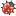eigs() bug

Joe 3 months ago updated by Pavel Holoborodko 2 months ago

I noticed a pretty severe bug for the eigs() function. In particular, if I do:

```A = sparse(mp(eye(4),34));
[evecs,evals] = eigs(A,1);```

I expect an identity matrix to be returned for both evecs and evals, however, I get the following result after one call of the above code:

```evecs =

0.6575264478049146996368762588811955
0.1350828341320901021606099191810989
0.09164029388649532440547542667314778
0.7355363042680420857014721043910981

evals =

1```

If I call it again I get an error:

```Error using mp/subsref (line 1375)
Index exceeds matrix dimensions.

Error in mpeigs (line 523)
D = ordeig(H(r,r));

Error in mp/eigs (line 3763)
[varargout{1:nargout}] = mpeigs(varargin{:});```

and in general it seems very sporadic, random, and most importantly, incorrect each time I call it. However, if I instead use the same function but without specifying the "1" as a parameter, e.g.:

```A = sparse(mp(eye(4),34));
[evecs,evals] = eigs(A);```

Everything seems to work as expected and I get identities returned for both evecs and evals. Obviously I don't need the eigenvalues and eigenvectors of the identity matrix but this is giving me little confidence in the eigenvectors and eigenvalues returned for the actual matrices I'm interested in. Am I missing something here or calling the function incorrectly? Also, I've noticed similar issues with the generalized eigenproblem equivalent of the above example.

Thank you.

﻿